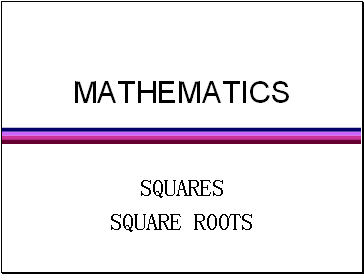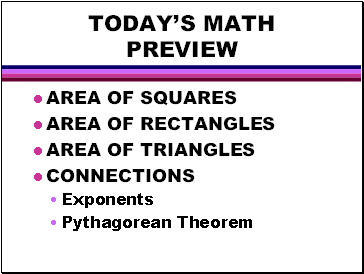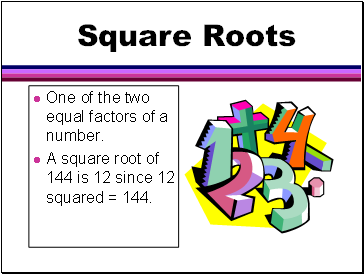# Squares and square rootsPage 1

#### WATCH ALL SLIDES

Slide 1MATHEMATICS

SQUARES

SQUARE ROOTS

Slide 2TODAY’S MATH PREVIEW

AREA OF SQUARES

AREA OF RECTANGLES

AREA OF TRIANGLES

CONNECTIONS

Exponents

Pythagorean Theorem

Slide 3## Math relations

Square Roots

Scientific Calculators

Slide 4## Area of Squares

Area formula:

A=s² (A=area & s=side)

S²means sxs

Slide 5## Area of Rectangles

Area formula:

A = l x w

L = length

W = width

Slide 6## Area of Triangles

Area formula:

A = ½ bh

B = base

H = height or altitude

Slide 7## Types of Triangles

Acute: triangles with angles less than 90º

Obtuse: triangles with one angle greater than 90º but less than 180º

Right: triangles with one angle equaling 90º

Slide 8## Connections

Exponents:

7² = 7 x 7

7² where 7 is the BASE and ² is the exponent.

Pythagorean Theorem:

A²+B²=C²

The square root of A & B are legs and the square root of C is the hypotenuse of the right triangle

Slide 9## Square Roots

One of the two equal factors of a number.

A square root of 144 is 12 since 12 squared = 144.

Slide 10## Scientific Calculator

Enter square root symbol before entering the number

Press enter or equal